```
0a
\$B0l(B0a1.1    \$B25(B0a1.5
\$BFs(B0a2.1    \$BF~(B0a2.3    \$BCz(B0a2.4    \$B<7(B0a2.13
\$B6e(B0a2.15
\$B;0(B0a3.1    \$B@n(B0a3.2    \$B<[(B0a3.5    \$B9)(B0a3.6
\$B5W(B0a3.7    \$BK|(B0a3.8    \$BK3(B0a3.11   \$B8J(B0a3.12
\$BM<(B0a3.14   \$B@#(B0a3.17   \$BBg(B0a3.18   \$B?O(B0a3.22
\$BM?(B0a3.23   \$B5Z(B0a3.24   \$B>f(B0a3.26   \$B:M(B0a3.27
\$B4](B0a3.28
\$BIT(B0a4.2    \$B6T(B0a4.3    \$B85(B0a4.5    \$B88(B0a4.6
\$BM=(B0a4.12   \$B8_(B0a4.15   \$BEM(B0a4.17   \$BB@(B0a4.18
\$B6'(B0a4.19   \$BE7(B0a4.21   \$BFb(B0a4.23   \$B;a(B0a4.25
\$B8^(B0a4.27   \$BIW(B0a4.31   \$B>#(B0a4.32   \$BLS(B0a4.33
\$BC0(B0a4.34   \$BFV(B0a4.35   \$BLh(B0a4.38   \$BCf(B0a4.40
\$BD\$(B0a4.41   \$B0f(B0a4.46
\$B0J(B0a5.1    \$BKL(B0a5.5    \$BL7(B0a5.6    \$B9*(B0a5.7
\$BJq(B0a5.9    \$B5V(B0a5.12   \$BFL(B0a5.13   \$B1z(B0a5.14
\$B3n(B0a5.15   \$BI,(B0a5.16   \$B@M(B0a5.18   \$BLp(B0a5.19
\$B:8(B0a5.20   \$BJ:(B0a5.21   \$B=P(B0a5.22   \$BL1(B0a5.23
\$BH>(B0a5.24   \$BK\(B0a5.25   \$BKv(B0a5.26   \$BL\$(B0a5.27
\$B<:(B0a5.28   \$B@8(B0a5.29   \$BJ[(B0a5.30   \$B4E(B0a5.32
\$B1{(B0a5.33   \$B9C(B0a5.34   \$BM3(B0a5.35   \$BJl(B0a5.36
\$B@\$(B0a5.37   \$B;K(B0a5.38   \$B?=(B0a5.39   \$B:}(B0a5.42
\$BB?(B0a6.5    \$B;`(B0a6.6    \$B5\$(B0a6.8    \$BN>(B0a6.11
\$B(B0a6.20   \$BMy(B0a6.22
\$BKh(B0a6.25   \$B:F(B0a6.26   \$B6J(B0a6.27
\$BNI(B0a7.3    \$B?H(B0a7.5    \$BMh(B0a7.6    \$B>5(B0a7.7
\$BB+(B0a7.8    \$BN\$(B0a7.9    \$B2f(B0a7.10   \$B99(B0a7.12
\$B0!(B0a7.14   \$Bh(B0a9.19
\$B4{(B0a10.5   \$B
\$B?M(B2a0.1
\$BJ)(B2a2.5    \$B2=(B2a2.6    \$B?N(B2a2.8    \$B2p(B2a2.9
\$B:#(B2a2.10
\$B@g(B2a3.1    \$B;E(B2a3.2    \$BBe(B2a3.3    \$BB>(B2a3.4
\$BIU(B2a3.6    \$BNa(B2a3.9
\$BIz(B2a4.1    \$B5Y(B2a4.2    \$B7o(B2a4.4    \$BH2(B2a4.5
\$BCg(B2a4.7    \$BG\$(B2a4.9    \$B6D(B2a4.10   \$BEA(B2a4.14
\$B2>(B2a4.15   \$BA4(B2a4.16   \$B4k(B2a4.17   \$B9g(B2a4.18
\$B2q(B2a4.19   \$BFy(B2a4.20
\$B0L(B2a5.1    \$B?-(B2a5.3    \$BH<(B2a5.4    \$BBN(B2a5.6
\$BGl(B2a5.7    \$B:4(B2a5.9    \$B:n(B2a5.10   \$B;w(B2a5.11
\$BC"(B2a5.14   \$BDc(B2a5.15   \$B=;(B2a5.19   \$B2?(B2a5.21
\$B;G(B2a5.23   \$BM>(B2a5.24   \$B4^(B2a5.25
\$B0M(B2a6.1    \$B;H(B2a6.2    \$B2A(B2a6.3    \$BNc(B2a6.7
\$B2B(B2a6.10   \$B;x(B2a6.11   \$B6!(B2a6.13   \$BJ;(B2a6.17
\$BIn(B2a6.20   \$Bu(B2b5.1    \$BNd(B2b5.3    \$B5a(B2b5.5
\$B=Z(B2b8.1    \$BE`(B2b8.2    \$B>-(B2b8.3
\$B6E(B2b14.1
\$B;R(B
\$B;R(B2c0.1    \$BN;(B2c0.3
\$B9&(B2c1.1
\$BB8(B2c3.1
\$B8I(B2c6.2
\$BB9(B2c7.1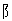\$BKI(B2d4.1    \$BK.(B2d4.7
\$BAK(B2d5.1    \$BIm(B2d5.4    \$Bc(B2d11.2   \$B1#(B2d11.3
\$BNY(B2d13.1
\$BRG(B
\$B0u(B2e4.1
\$BB((B2e5.1    \$BMq(B2e5.2    \$B5Q(B2e5.3
\$B27(B2e7.1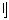\$BEa(B2f0.1
\$B4"(B2f2.1    \$B@Z(B2f2.2
\$B4)(B2f3.1    \$B>\$(B2f3.3
\$B=#(B2f4.1    \$B7:(B2f4.2    \$BNs(B2f4.4
\$BH=(B2f5.2    \$BJL(B2f5.3
\$B@)(B2f6.1    \$B;I(B2f6.2    \$BE~(B2f6.4    \$B9o(B2f6.7
\$B:~(B2f6.9    \$B7t(B2f6.10
\$B:o(B2f7.4    \$B7@(B2f7.6
\$BK6(B2f8.1    \$B7u(B2f8.5    \$B:^(B2f8.6    \$B9d(B2f8.7
\$B5"(B2f8.8
\$B>j(B2f9.1    \$BI{(B2f9.2
\$B3d(B2f10.1   \$BAO(B2f10.3
\$B7`(B2f13.2
\$BNO(B
\$BNO(B2g0.1
\$B2C(B2g3.1    \$B8y(B2g3.2    \$BMD(B2g3.3
\$B=u(B2g5.1    \$BNe(B2g5.4    \$BEX(B2g5.6
\$B3/(B2g6.1    \$B8z(B2g6.2
\$BD<(B2g7.1    \$BM&(B2g7.3
\$B6<(B2g8.2
\$BF0(B2g9.1    \$B4*(B2g9.3
\$B6P(B2g10.1
\$B4+(B2g11.1   \$B@*(B2g11.6
\$BKt(B
\$BKt(B2h0.1
\$BAP(B2h2.1    \$B<}(B2h2.2    \$BM'(B2h2.3
\$BHi(B2h3.1
\$B=G(B2h6.1    \$Bi(B2i2.1
\$B&(B2j9.7
\$BHZ(B2j10.1
\$BN"(B2j11.2   \$B4~(B2j11.5
\$B9k(B2j12.3
\$BK+(B2j13.1
\$B==(B
\$B==(B2k0.1
\$B43(B2k1.1    \$B@i(B2k1.2
\$B;Y(B2k2.1    \$B8a(B2k2.2
\$B8E(B2k3.1    \$BJ?(B2k3.4
\$B9'(B2k4.3    \$B9M(B2k4.4    \$BO7(B2k4.5    \$B4L(B2k4.6
\$B9n(B2k5.1
\$B6((B2k6.1    \$BD>(B2k6.2    \$BK[(B2k6.5
\$BFn(B2k7.1
\$B??(B2k8.1    \$B:w(B2k8.2
\$BGn(B2k10.1
\$B=`(B2k11.1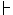\$B>e(B2m1.1    \$B2<(B2m1.2
\$B;_(B2m2.2
\$B30(B2m3.1    \$B@j(B2m3.2    \$B@5(B2m3.3    \$BHf(B2m3.5
\$BBn(B2m6.2
\$BDg(B2m7.1    \$BE@(B2m7.2    \$B5T(B2m7.3
\$B5u(B2m9.1
\$B6s(B2m11.1   \$BN:(B2m11.2
\$B5?(B2m12.1
\$BIf(B2m13.1   \$BN8(B2m13.2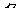\$B?'(B2n4.1    \$BAh(B2n4.2    \$B4m(B2n4.3
\$B3Q(B2n5.1
\$BLH(B2n6.1
\$BIi(B2n7.1    \$B5^(B2n7.2
\$BJY(B2n8.1
\$B>](B2n10.1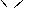\$BH,(B2o0.1
\$BJ,(B2o2.1    \$B8x(B2o2.2    \$BIc(B2o2.3
\$BMS(B2o4.1
\$BDo(B2o5.1    \$BC+(B2o5.3    \$BJ<(B2o5.6    \$B8b(B2o5.7
\$BJB(B2o6.1    \$BE5(B2o6.5
\$B
\$BJU(B2q2.1    \$B9~(B2q2.3
\$B=d(B2q3.3    \$B?W(B2q3.5
\$BDn(B2q4.2    \$B6a(B2q4.3    \$B7^(B2q4.4    \$BJV(B2q4.5
\$BE3(B2q5.2    \$B=R(B2q5.3    \$B1d(B2q5.4    \$BGw(B2q5.5
\$BLB(B2q6.1    \$B7z(B2q6.2    \$BB`(B2q6.3    \$BDI(B2q6.4
\$BF((B2q6.5    \$B5U(B2q6.8    \$BAw(B2q6.9
\$BO"(B2q7.2    \$BB.(B2q7.4    \$BD~(B2q7.5    \$BC`(B2q7.6
\$B@B(B2q7.8    \$BF)(B2q7.10   \$BB\$(B2q7.11   \$BES(B2q7.16
\$BDL(B2q7.18
\$B?J(B2q8.1    \$BBa(B2q8.2    \$BM7(B2q8.3    \$B0o(B2q8.6
\$B=5(B2q8.7
\$B6x(B2q9.1    \$BC#(B2q9.8    \$B1?(B2q9.10   \$B?k(B2q9.13
\$BF;(B2q9.14   \$BJW(B2q9.16   \$BCY(B2q9.17   \$B2a(B2q9.18
\$B8/(B2q10.2   \$B1s(B2q10.4   \$B0c(B2q10.5
\$BAx(B2q11.2   \$BE,(B2q11.3   \$B
\$BK^(B2s1.1
\$BIw(B2s7.1    \$BCJ(B2s7.2
\$BR9(B
\$B6h(B2t2.1    \$B5p(B2t2.2    \$BI\$(B2t2.3
\$B>"(B2t4.2    \$B?C(B2t4.3
\$B0e(B2t5.2
\$B2%(B2t6.1
\$BF?(B2t8.2
\$BNW(B2t15.1\$B?e(B3a0.1
\$B1J(B3a1.1    \$BI9(B3a1.2
\$B=A(B3a2.1
\$BCS(B3a3.4    \$B1x(B3a3.5    \$B4@(B3a3.6    \$B9>(B3a3.8
\$B2-(B3a4.5    \$B7h(B3a4.6    \$BD@(B3a4.9    \$BKW(B3a4.15
\$B5%(B3a4.16   \$BBt(B3a4.18
\$B5c(B3a5.1    \$BJ((B3a5.3    \$BL}(B3a5.6    \$BGH(B3a5.9
\$BHg(B3a5.10   \$B1K(B3a5.14   \$BGq(B3a5.15   \$BCm(B3a5.16
\$BK"(B3a5.18   \$BK!(B3a5.20   \$B67(B3a5.21   \$B1h(B3a5.23
\$B>B(B3a5.24   \$B<#(B3a5.28   \$BE%(B3a5.29   \$B2O(B3a5.30
\$B@t(B3a5.33   \$BBY(B3a5.34
\$BDE(B3a6.1    \$B@u(B3a6.4    \$BIb(B3a6.11   \$B@v(B3a6.12
\$B9?(B3a6.14   \$B3h(B3a6.16   \$B>t(B3a6.18   \$BMN(B3a6.19
\$B3\$(B3a6.20   \$BGI(B3a6.21   \$BF6(B3a6.25
\$BC(B3a7.16   \$B?;(B3a7.17   \$BMa(B3a7.18
\$BN^(B3a7.21
\$B=J(B3a8.5    \$B3i(B3a8.13   \$B:.(B3a8.14   \$BC8(B3a8.15
\$B7L(B3a8.16   \$B@6(B3a8.18   \$B=B(B3a8.19   \$B>D(B3a8.20
\$B?<(B3a8.21   \$BE:(B3a8.22   \$B1U(B3a8.29   \$B:Q(B3a8.30
\$BNC(B3a8.31   \$B36(B3a8.33
\$BB,(B3a9.4    \$B8P(B3a9.8    \$B9A(B3a9.13   \$BOQ(B3a9.15
\$B29(B3a9.21   \$B<>(B3a9.22   \$BEr(B3a9.23   \$BK~(B3a9.25
\$B<"(B3a9.27   \$BEO(B3a9.35   \$B12(B3a9.36   \$B8:(B3a9.37
\$B3j(B3a10.6   \$BBl(B3a10.8   \$B9B(B3a10.9   \$BBZ(B3a10.14
\$BMO(B3a10.15  \$B4A(B3a10.17  \$BGy(B3a10.18  \$B8;(B3a10.25
\$BLG(B3a10.26
\$B5y(B3a11.1   \$BA2(B3a11.2   \$BI:(B3a11.9   \$B
\$BEZ(B3b0.1
\$B5n(B3b2.2
\$BCO(B3b3.1    \$B;{(B3b3.5    \$B;j(B3b3.6    \$B@h(B3b3.7
\$B:_(B3b3.8
\$BK7(B3b4.1    \$B9#(B3b4.6    \$B:d(B3b4.7    \$B6Q(B3b4.8
\$BAv(B3b4.9    \$B@V(B3b4.10
\$BDZ(B3b5.4    \$B9,(B3b5.9
\$B>k(B3b6.1    \$B3@(B3b6.5    \$B7?(B3b6.11   \$BIu(B3b6.13
\$BIk(B3b6.14
\$BKd(B3b7.2    \$B5/(B3b7.11
\$B0h(B3b8.3    \$BG](B3b8.6    \$BKY(B3b8.11   \$B4p(B3b8.12
\$BBD(B3b8.14   \$B<9(B3b8.15
\$B4.(B3b9.1    \$B>l(B3b9.6    \$BDi(B3b9.7    \$BEc(B3b9.9
\$BDM(B3b9.10   \$BJ=(B3b9.11   \$B7x(B3b9.13   \$BJs(B3b9.16
\$BD6(B3b9.18   \$BAS(B3b9.20
\$B2t(B3b10.3   \$B1v(B3b10.5   \$B=N(B3b10.8   \$BA:(B3b10.9
\$BEI(B3b10.10
\$B6-(B3b11.1   \$BA}(B3b11.3   \$BKO(B3b11.4   \$BDF(B3b11.7
\$BJ/(B3b12.1   \$BJ^(B3b12.4
\$B2u(B3b13.3   \$B>m(B3b13.4   \$BCE(B3b13.5   \$B:&(B3b13.6
\$BJI(B3b13.7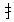\$B6(B3c4.11   \$BM^(B3c4.12   \$BHc(B3c4.13   \$B93(B3c4.15
\$B5;(B3c4.16   \$BEj(B3c4.18   \$BBr(B3c4.21
\$BBs(B3c5.1    \$BGR(B3c5.3    \$B2!(B3c5.5    \$BCj(B3c5.7
\$BKu(B3c5.9    \$B@[(B3c5.11   \$BHd(B3c5.13   \$BGo(B3c5.14
\$BJz(B3c5.15   \$BDq(B3c5.18   \$BC4(B3c5.20   \$B2}(B3c5.21
\$B>7(B3c5.22   \$B3H(B3c5.25   \$B5r(B3c5.26   \$B94(B3c5.28
\$B5q(B3c5.29
\$B64(B3c6.1    \$B9i(B3c6.2    \$BD)(B3c6.5    \$B;}(B3c6.8
\$B3g(B3c6.12   \$B=&(B3c6.14   \$B;X(B3c6.15
\$BA^(B3c7.2    \$BJa(B3c7.3    \$BA\(B3c7.6    \$B?6(B3c7.15
\$B?d(B3c8.1    \$B3](B3c8.6    \$BGS(B3c8.8    \$B@\(B3c8.10
\$B95(B3c8.11   \$B7G(B3c8.13   \$B:N(B3c8.14   \$B(B3d3.12
\$B?a(B3d4.3    \$B6c(B3d4.8    \$BDh(B3d4.14   \$B9p(B3d4.18
\$BH](B3d4.20   \$BMp(B3d4.21   \$BF&(B3d4.22   \$B7/(B3d4.23
\$BL#(B3d5.3    \$B8F(B3d5.4    \$BCN(B3d5.14   \$B4q(B3d5.17
\$B:i(B3d6.12   \$BIJ(B3d6.15
\$B:6(B3d7.8    \$B0w(B3d7.10   \$B?0(B3d7.12   \$BE/(B3d7.13
\$BM#(B3d8.1    \$B3e(B3d8.8    \$B>'(B3d8.9    \$B7<(B3d8.17
\$B5J(B3d9.7    \$B4-(B3d9.19   \$B="(B3d9.21   \$BEP(B3d9.26
\$BC;(B3d9.27   \$B?R(B3d9.29
\$BC2(B3d10.8   \$B;L(B3d10.13  \$B72(B3d10.14  \$BK-(B3d10.15
\$BLD(B3d11.1
\$BJ.(B3d12.8   \$B>|(B3d12.11  \$B4o(B3d12.13
\$B3E(B3d14.1   \$B6:(B3d14.5
\$B=w(B
\$B=w(B3e0.1
\$B9%(B3e2.1    \$BE[(B3e2.2
\$BG!(B3e3.1    \$BH^(B3e3.2
\$BK8(B3e4.1    \$BG%(B3e4.3    \$BL/(B3e4.5    \$BBE(B3e4.9
\$B@+(B3e5.3    \$BKe(B3e5.4    \$B;P(B3e5.8    \$B;O(B3e5.9
\$B:J(B3e5.10
\$B0y(B3e6.8    \$B;Q(B3e6.10   \$BMW(B3e6.11
\$BL<(B3e7.2    \$B8d(B3e7.3    \$B?1(B3e7.10   \$BI1(B3e7.11
\$B:'(B3e8.4    \$BIX(B3e8.6    \$BGL(B3e8.9
\$BG^(B3e9.2    \$BL;(B3e9.3
\$B2G(B3e10.6   \$B7y(B3e10.7
\$BCd(B3e11.5
\$B>n(B3e13.1
\$B6R(B
\$BI[(B3f2.1
\$BHA(B3f3.1
\$B4u(B3f4.1
\$B?c(B3f6.1
\$BBS(B3f7.1    \$B;U(B3f7.2
\$BD"(B3f8.2
\$BK9(B3f9.1    \$BI}(B3f9.2
\$BJ>(B3f12.4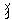\$B8\$(B3g0.1
\$BHH(B3g2.1
\$B68(B3g4.2
\$BFH(B3g6.1    \$B69(B3g6.2    \$B(B3i7.3
\$B=Q(B3i8.2    \$BF@(B3i8.4
\$B8f(B3i9.1    \$B39(B3i9.2    \$BI|(B3i9.4    \$B=[(B3i9.6
\$BHy(B3i10.1
\$BD'(B3i11.2   \$BFA(B3i11.3
\$B>W(B3i12.1   \$BE0(B3i12.2
\$B9U(B3i13.1   \$B1R(B3i13.3
\$BWD(B
\$B7A(B3j4.1
\$B;2(B3j5.1
\$B:L(B3j8.1    \$BD&(B3j8.2
\$B>4(B3j11.1   \$BH1(B3j11.3
\$B1F(B3j12.1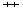\$Bx(B3k9.19   \$BMU(B3k9.21
\$BJg(B3k9.23
\$BL4(B3k10.14  \$BC_(B3k10.16  \$BJh(B3k10.18  \$BKk(B3k10.19
\$B7\$(B3k10.34
\$BJi(B3k11.12  \$BJk(B3k11.14
\$BB"(B3k12.17
\$B?E(B3k13.3   \$BGv(B3k13.11  \$BLt(B3k13.15  \$B70(B3k13.17
\$BA&(B3k13.25
\$BHM(B3k15.4   \$BKz(B3k15.7
\$BAt(B3k16.8
\$BU_(B
\$B;z(B3m2.1    \$B7j(B3m2.2
\$B0B(B3m3.1    \$B,(B3m7.7    \$BMF(B3m7.8
\$B
\$B>.(B3n0.1
\$B>/(B3n1.1
\$B8w(B3n3.2    \$BEv(B3n3.3    \$BNt(B3n3.4
\$B>S(B3n4.1    \$B3X(B3n4.2    \$BO+(B3n4.3    \$BF}(B3n4.4
\$B>0(B3n5.2    \$BJb(B3n5.3
\$B1I(B3n6.1    \$BC1(B3n6.2    \$B8)(B3n6.3
\$B5s(B3n7.1    \$BE^(B3n7.2
\$BAc(B3n8.1    \$B7V(B3n8.2    \$B>o(B3n8.3    \$BF2(B3n8.4
\$B1D(B3n9.2    \$B3P(B3n9.3    \$B>8(B3n9.4
\$BM@(B3n10.1   \$B>)(B3n10.4
\$B>^(B3n12.1
\$B87(B3n14.1
\$B;3(B
\$B;3(B3o0.1
\$B4t(B3o4.1
\$BL((B3o5.4    \$B4d(B3o5.10   \$B4_(B3o5.11   \$B3Y(B3o5.12
\$B6.(B3o6.1    \$BF=(B3o6.3    \$BC:(B3o6.5    \$BM)(B3o6.6
\$BJv(B3o7.6    \$BEg(B3o7.9
\$B:j(B3o8.3    \$BJx(B3o8.7    \$B?r(B3o8.9
\$B;N(B
\$B;N(B3p0.1
\$B5H(B3p3.1
\$B;V(B3p4.1    \$B0m(B3p4.2    \$BGd(B3p4.3    \$B@<(B3p4.4
\$B3L(B3p8.1
\$B4n(B3p9.1
\$B8](B3p10.2
\$BVx(B
\$B9-(B3q2.1    \$BD#(B3q2.2
\$B>2(B3q4.1    \$B1~(B3q4.2    \$B=x(B3q4.4
\$BI\(B3q5.2    \$BDl(B3q5.3    \$BE9(B3q5.4
\$BEY(B3q6.1    \$BDm(B3q6.3
\$B8K(B3q7.1    \$B:B(B3q7.2    \$BEb(B3q7.3    \$B@J(B3q7.4
\$B9/(B3q8.1    \$BMG(B3q8.2    \$BKc(B3q8.3    \$BO-(B3q8.4
\$B=n(B3q8.7
\$BGQ(B3q9.3
\$BNw(B3q10.1
\$BIe(B3q11.3
\$BK`(B3q12.6    \$B7D(B3q12.8
\$BKa(B3q13.3
\$BNo(B3q16.5
\$BKb(B3q18.2
\$B<\(B3r1.1
\$BFt(B3r2.2
\$B?T(B3r3.1
\$BG"(B3r4.1    \$BHx(B3r4.2    \$B6I(B3r4.4
\$BFO(B3r5.1    \$B6~(B3r5.2    \$B5o(B3r5.3
\$B20(B3r6.3
\$BE8(B3r7.2
\$BB0(B3r9.1
\$BEB(B3r10.1
\$BAX(B3r11.2
\$BMz(B3r12.1
\$BSx(B
\$B<|(B3s2.1    \$B;M(B3s2.2
\$B2s(B3s3.1    \$B0x(B3s3.2    \$BCD(B3s3.3
\$B:\$(B3s4.1    \$B0O(B3s4.2    \$B?^(B3s4.3
\$B9q(B3s5.1    \$B8G(B3s5.2
\$BLL(B3s6.1
\$B7w(B3s9.1
\$B1`(B3s10.1
\$BLZ(B
\$BLZ(B4a0.1
\$B;%(B4a1.1
\$BKQ(B4a2.3    \$B4y(B4a2.4    \$B5`(B4a2.6
\$B?y(B4a3.2    \$B:`(B4a3.6    \$BB<(B4a3.10
\$BNS(B4a4.1    \$BKg(B4a4.4    \$BGU(B4a4.11   \$B@O(B4a4.12
\$B>>(B4a4.16   \$B;^(B4a4.18   \$BOH(B4a4.19   \$BHD(B4a4.21
\$B?u(B4a4.22
\$BAj(B4a5.3    \$BJA(B4a5.9    \$BCl(B4a5.12   \$BLx(B4a5.17
\$B8O(B4a5.26   \$B::(B4a5.32   \$BK?(B4a5.33   \$B=@(B4a5.34
\$B@w(B4a5.35   \$B2M(B4a5.36
\$B;7(B4a6.1    \$B3t(B4a6.3    \$B:,(B4a6.5    \$BEm(B4a6.10
\$B:y(B4a6.15   \$B3J(B4a6.17   \$B3K(B4a6.22   \$B9;(B4a6.24
\$B@r(B4a6.26   \$BG_(B4a6.27   \$B;&(B4a6.35
\$B3#(B4a7.23
\$BEo(B4a8.3    \$BC*(B4a8.10   \$B6K(B4a8.11   \$B4}(B4a8.14
\$BK@(B4a8.20   \$B4=(B4a8.25   \$B8!(B4a8.28   \$B?"(B4a8.32
\$B?9(B4a8.39
\$BO0(B4a9.10   \$B3Z(B4a9.29
\$B35(B4a10.2   \$B9=(B4a10.10  \$BLO(B4a10.16  \$BMM(B4a10.25
\$BAe(B4a11.7   \$BI8(B4a11.8   \$B2#(B4a11.13  \$B8"(B4a11.18
\$B5!(B4a12.1   \$B(B4b7.7    \$BC&(B4b7.8
\$BD1(B4b8.1    \$B>!(B4b8.4    \$BOS(B4b8.6    \$B4|(B4b8.11
\$BD+(B4b8.12
\$B9x(B4b9.3    \$BJ"(B4b9.4    \$BD2(B4b9.8
\$BKl(B4b10.6   \$B@E(B4b10.9
\$BKD(B4b12.1
\$BF%(B4b13.1
\$B?q(B4b14.3
\$BB!(B4b15.2   \$BGF(B4b15.4
\$BF-(B4b16.3
\$BF|(B
\$BF|(B4c0.1
\$B5l(B4c1.1    \$BGr(B4c1.3
\$BAa(B4c2.1    \$B;](B4c2.2    \$BI4(B4c2.3    \$B=\(B4c2.5
\$B;y(B4c3.3
\$BL@(B4c4.1    \$B>:(B4c4.5    \$B0W(B4c4.9    \$B:+(B4c4.10
\$BE*(B4c4.12   \$B<(B4c5.4    \$BKA(B4c5.6
\$B@1(B4c5.7    \$B@'(B4c5.9    \$B=U(B4c5.13   \$B3'(B4c5.14
\$BCk(B4c5.15
\$B;~(B4c6.2    \$B=q(B4c6.6    \$B=^(B4c6.9
\$BAb(B4c7.10   \$B=,(B4c7.11   \$B4%(B4c7.14
\$B6G(B4c8.1    \$B@2(B4c8.2    \$BHU(B4c8.3    \$B=k(B4c8.5
\$B>=(B4c8.6    \$B7J(B4c8.8    \$BNL(B4c8.9    \$B:G(B4c8.10
\$BBX(B4c8.12
\$B2K(B4c9.1    \$B0E(B4c9.2    \$BCH(B4c9.4    \$B44(B4c9.8
\$BK=(B4c11.2   \$B;C(B4c11.3
\$BF^(B4c12.1
\$BMK(B4c14.1   \$BJ\$(B4c14.6
\$B6A(B4c15.3
\$B2P(B
\$B2P(B4d0.1
\$BEt(B4d2.1
\$B:R(B4d3.3
\$B?f(B4d4.1    \$BO'(B4d4.2    \$B1j(B4d4.4
\$BH*(B4d5.1    \$B0Y(B4d5.8
\$BNu(B4d6.3
\$B9u(B4d7.2
\$B>F(B4d8.4    \$BL5(B4d8.8    \$BH(B4d9.12
\$B=O(B4d10.5
\$B7.(B4d11.3   \$BG.(B4d11.4   \$BL[(B4d11.5
\$BG3(B4d12.2
\$BAg(B4d13.6
\$BGz(B4d15.2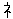\$B<((B4e0.1
\$BNi(B4e1.1
\$BM(B4e6.1    \$BI<(B4e6.2    \$B:W(B4e6.3    \$B0S(B4e6.4
\$B;k(B4e7.1
\$B6X(B4e8.3
\$BJ!(B4e9.1    \$BA5(B4e9.2    \$B2R(B4e9.4
\$BNl(B4e11.1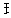\$B2&(B4f0.1    \$B6L(B4f0.2
\$B(B4f7.6
\$B6W(B4f8.11
\$B@;(B4f9.9
\$B4D(B4f13.1
\$B<%(B4f14.2
\$B5m(B
\$B5m(B4g0.1
\$BKR(B4g4.1    \$BJ*(B4g4.2
\$B@7(B4g5.1
\$BFC(B4g6.1
\$B2r(B4g9.1
\$B5>(B4g13.1
\$BJ}(B
\$BJ}(B4h0.1
\$BJ|(B4h4.1
\$B;\(B4h5.1
\$BN9(B4h6.4
\$B@{(B4h7.2    \$BB2(B4h7.3
\$B4z(B4h10.1
\$BTi(B
\$BE_(B4i2.1    \$B=h(B4i2.2
\$B2~(B4i3.1    \$B96(B4i3.2    \$B3F(B4i3.3
\$B>r(B4i4.1    \$BG~(B4i4.2
\$B@/(B4i5.1    \$B8N(B4i5.2
\$B65(B4i6.1    \$BCW(B4i6.2    \$BIR(B4i6.3
\$B5_(B4i7.1    \$B(B4j8.2
\$B2N(B4j10.2
\$B4?(B4j11.1
\$B?4(B
\$B?4(B4k0.1
\$BK;(B4k3.2    \$BG&(B4k3.3    \$B4w(B4k3.4
\$B2w(B4k4.2    \$BCi(B4k4.6
\$B@-(B4k5.4    \$BI](B4k5.6    \$B2x(B4k5.11   \$BE\(B4k5.19
\$BBU(B4k5.21
\$B:((B4k6.2    \$B91(B4k6.5    \$B2y(B4k6.12   \$B7C(B4k6.16
\$BB)(B4k6.17   \$B62(B4k6.19   \$B28(B4k6.23
\$B8g(B4k7.5    \$BG:(B4k7.11   \$B1Y(B4k7.15   \$B0-(B4k7.17
\$B45(B4k7.18   \$BM*(B4k7.20
\$B;4(B4k8.5    \$B>p(B4k8.9    \$B@K(B4k8.11   \$BEi(B4k8.13
\$BOG(B4k8.16   \$BHa(B4k8.18
\$BBF(B4k9.6    \$B92(B4k9.10   \$BL{(B4k9.13   \$B6r(B4k9.15
\$B=%(B4k9.16   \$BA[(B4k9.18   \$B46(B4k9.21
\$B34(B4k10.2   \$B?5(B4k10.3   \$BBV(B4k10.13
\$BA~(B4k11.7   \$BK}(B4k11.8   \$B47(B4k11.9   \$B0V(B4k11.13
\$BJ0(B4k12.6   \$B7F(B4k12.10
\$B48(B4k13.3   \$B21(B4k13.5   \$B2{(B4k13.9   \$B:)(B4k13.12
\$BD((B4k14.3
\$B7|(B4k16.2
\$B8M(B
\$B8M(B4m0.1
\$BLa(B4m3.1
\$B8*(B4m4.1    \$BK<(B4m4.2    \$B=j(B4m4.3
\$B@p(B4m6.1
\$B8[(B4m8.1
\$BHb(B4m8.2
\$BXy(B
\$B@.(B4n2.1
\$B2|(B4n3.1    \$B<0(B4n3.2    \$BFu(B4n3.3
\$B0R(B4n5.2    \$BIp(B4n5.3
\$B:O(B4n6.1
\$B1[(B4n8.2    \$B4v(B4n8.4
\$B@o(B4n9.2    \$B:P(B4n9.5
\$B5:(B4n11.1
\$B@P(B
\$B@P(B5a0.1
\$B8&(B5a4.1    \$B:=(B5a4.3    \$B:U(B5a4.6
\$BGK(B5a5.1    \$BK\$(B5a5.3
\$B9E(B5a7.1    \$BN2(B5a7.3    \$B>K(B5a7.6
\$B8k(B5a8.9
\$BHj(B5a9.2    \$B<'(B5a9.6
\$B3N(B5a10.3
\$B>L(B5a12.2
\$BAC(B5a13.2
\$BN)(B
\$BN)(B5b0.1
\$B?I(B5b2.2
\$B2;(B5b4.3
\$BN5(B5b5.3
\$B>O(B5b6.3    \$B;:(B5b6.4    \$BMb(B5b6.6
\$BF8(B5b7.3
\$B0U(B5b8.2    \$B?7(B5b8.3    \$B<-(B5b8.4
\$BC<(B5b9.2
\$B?F(B5b11.1
\$B6%(B5b15.1
\$BL\(B
\$BL\(B5c0.1
\$B<+(B5c1.1
\$B8+(B5c2.1
\$B6q(B5c3.1
\$B=-(B5c4.3    \$B4G(B5c4.4    \$B>J(B5c4.7    \$B=b(B5c4.8
\$BL2(B5c5.2
\$B4c(B5c6.1    \$BD/(B5c6.2    \$B5,(B5c6.9
\$B?#(B5c7.4
\$B?g(B5c8.2    \$BFD(B5c8.9
\$BF3(B5c9.3
\$BMw(B5c12.7
\$B=V(B5c13.1   \$B4Q(B5c13.7
\$B2S(B
\$BMx(B5d2.1    \$B;d(B5d2.2    \$B5((B5d2.3    \$B=((B5d2.4
\$BOB(B5d3.1    \$B0Q(B5d3.2
\$B=)(B5d4.1    \$BIC(B5d4.2    \$B2J(B5d4.3    \$B9a(B5d4.5
\$BCa(B5d5.2    \$BHk(B5d5.6    \$BAE(B5d5.7    \$B>N(B5d5.8
\$B0\(B5d6.1
\$BDx(B5d7.2    \$B@G(B5d7.4
\$BCU(B5d8.1
\$B
\$B0a(B5e0.1
\$B=i(B5e2.1
\$BHo(B5e5.3    \$BB^(B5e5.11
\$BNv(B5e6.7    \$BAu(B5e6.8    \$B:[(B5e6.9
\$BJd(B5e7.1    \$BM5(B5e7.3
\$BMg(B5e8.1    \$B3l(B5e8.7    \$B@=(B5e8.9
\$BJ#(B5e9.3
\$B6_(B5e13.2
\$B=1(B5e16.2
\$BED(B
\$BED(B5f0.1
\$BD.(B5f2.1    \$BCK(B5f2.2
\$B0_(B5f4.3    \$B;W(B5f4.4    \$B3&(B5f4.7    \$BH\(B5f4.8
\$BHJ(B5f5.1    \$BN1(B5f5.4    \$B@&(B5f5.5    \$B54(B5f5.6
\$BN,(B5f6.4    \$BN_(B5f6.5    \$B0[(B5f6.7
\$BN](B5f7.2    \$B>v(B5f7.3    \$BHV(B5f7.4
\$B:2(B5f9.2    \$BI!(B5f9.3
\$BL%(B5f10.1
\$BJ3(B5f11.2\$BGc(B5g7.2
\$B=p(B5g8.1    \$B:a(B5g8.4    \$BCV(B5g8.8
\$BH3(B5g9.1
\$BHm(B5g10.2
\$B<_(B5g12.1
\$BMe(B5g14.1
\$B;.(B
\$B;.(B5h0.1
\$B7l(B5h1.1
\$B@9(B5h6.1    \$BEp(B5h6.2
\$B=0(B5h7.1
\$BLA(B5h8.1
\$B4F(B5h10.1   \$BHW(B5h10.2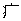\$B1V(B5i4.2
\$BHh(B5i5.2    \$BIB(B5i5.3    \$B>I(B5i5.4    \$B<@(B5i5.12
\$BN!(B5i7.2    \$BDK(B5i7.7    \$BEw(B5i7.8
\$BCT(B5i8.1
\$BNE(B5i12.3
\$BJJ(B5i13.2   \$BL~(B5i13.3
\$B;e(B
\$B;e(B6a0.1
\$B7O(B6a1.1
\$B5i(B6a3.2    \$B5j(B6a3.4    \$B5*(B6a3.5    \$B9H(B6a3.6
\$BLs(B6a3.7
\$BKB(B6a4.1    \$B=c(B6a4.3    \$B;f(B6a4.4    \$BG<(B6a4.5
\$BJ6(B6a4.8    \$BLf(B6a4.9    \$BAG(B6a4.12
\$B:Y(B6a5.1    \$B?B(B6a5.2    \$B:0(B6a5.5    \$BAH(B6a5.7
\$B=*(B6a5.9    \$B>R(B6a5.10   \$B7P(B6a5.11
\$B7k(B6a6.5    \$BMm(B6a6.6    \$B5k(B6a6.7    \$B3((B6a6.8
\$B9J(B6a6.9    \$BE}(B6a6.10   \$B@d(B6a6.11   \$B;g(B6a6.15
\$B8((B6a7.3    \$BB3(B6a7.5    \$B7Q(B6a7.8
\$B0](B6a8.1    \$BN}(B6a8.2    \$B=o(B6a8.3    \$BLJ(B6a8.8
\$BNP(B6a8.15   \$BAm(B6a8.20   \$B9K(B6a8.23   \$BLV(B6a8.25
\$BFl(B6a9.1    \$B@~(B6a9.7    \$B4K(B6a9.8    \$B1o(B6a9.10
\$BDy(B6a9.11   \$BJT(B6a9.13   \$BK%(B6a9.15   \$B6[(B6a9.17
\$B=D(B6a10.2   \$BG{(B6a10.3   \$B0^(B6a10.7   \$BHK(B6a10.13
\$BA!(B6a11.1   \$B@S(B6a11.8   \$B=L(B6a11.9
\$BA6(B6a12.2   \$B?%(B6a12.6
\$B7+(B6a13.3
\$BJF(B
\$BJF(B6b0.1
\$BNA(B6b4.4    \$B?h(B6b4.5    \$BJ4(B6b4.6
\$BN3(B6b5.1    \$BAF(B6b5.2    \$BG4(B6b5.4    \$BQ(B6b6.1    \$B1|(B6b6.9    \$B;u(B6b6.11
\$B@:(B6b8.1
\$BE|(B6b10.3
\$BNp(B6b11.6
\$BNH(B6b12.1   \$BK](B6b12.3
\$B=.(B
\$B=.(B6c0.1
\$B9R(B6c4.2    \$BHL(B6c4.3
\$BGu(B6c5.2    \$BA%(B6c5.4
\$BDz(B6c6.2
\$B4O(B6c15.2
\$BCn(B
\$BCn(B6d0.1
\$B2c(B6d4.5    \$B;=(B6d4.8
\$BP(B6f4.1
\$BBh(B6f5.5    \$BE+(B6f5.6    \$BId(B6f5.12
\$BI.(B6f6.1    \$B:v(B6f6.2    \$B6Z(B6f6.4    \$BEy(B6f6.9
\$BEz(B6f6.12   \$BE{(B6f6.15
\$B@a(B6f7.3
\$B;;(B6f8.7    \$B4I(B6f8.12   \$B2U(B6f8.15
\$BHO(B6f9.3    \$BH"(B6f9.4
\$BFF(B6f10.1   \$BC[(B6f10.5
\$B4J(B6f12.5
\$BJm(B6f13.4
\$B@R(B6f14.1
\$B8@(B
\$B8@(B7a0.1
\$B7W(B7a2.1    \$BD{(B7a2.3
\$BBw(B7a3.1    \$BF\$(B7a3.3    \$B5-(B7a3.5    \$B71(B7a3.6
\$BK,(B7a4.1    \$B5v(B7a4.3    \$B>Y(B7a4.6    \$B@_(B7a4.7
\$BLu(B7a4.8
\$BAJ(B7a5.2    \$BI>(B7a5.3    \$B>Z(B7a5.5    \$B:>(B7a5.6
\$B?G(B7a5.9    \$B>[(B7a5.10   \$B1S(B7a5.14   \$B;l(B7a5.15
\$B@?(B7a6.3    \$B;m(B7a6.5    \$B5M(B7a6.7    \$BOC(B7a6.8
\$B8X(B7a6.9    \$B3:(B7a6.10   \$B>\(B7a6.12   \$B;n(B7a6.18
\$B8m(B7a7.2    \$BM6(B7a7.4    \$B8l(B7a7.6    \$B;o(B7a7.8
\$BFI(B7a7.9    \$BG'(B7a7.10   \$B@b(B7a7.12   \$BCB(B7a7.15
\$B@@(B7a7.17
\$B2](B7a8.2    \$B=t(B7a8.3    \$B1Z(B7a8.6    \$BCL(B7a8.7
\$B@A(B7a8.8    \$BBz(B7a8.10   \$BO@(B7a8.13   \$BD4(B7a8.16
\$B;p(B7a9.4    \$BKE(B7a9.8    \$BMX(B7a9.9    \$BM!(B7a9.13
\$By(B7a13.1   \$B8n(B7a13.3   \$B5D(B7a13.4
\$B3-(B
\$B3-(B7b0.1
\$BB'(B7b2.1
\$B:b(B7b3.1    \$B9W(B7b3.3
\$BGT(B7b4.1    \$BHN(B7b4.2    \$B4S(B7b4.3    \$B@U(B7b4.4
\$B2_(B7b4.5
\$BCy(B7b5.1    \$BHq(B7b5.4    \$B5.(B7b5.7    \$BKG(B7b5.8
\$BB_(B7b5.9    \$B2l(B7b5.10
\$BOE(B7b6.1    \$BB1(B7b6.3    \$BDB(B7b6.6    \$B;q(B7b6.7
\$BGe(B7b8.1    \$B;r(B7b8.2    \$BIj(B7b8.4    \$B;?(B7b8.6
\$B(B7c4.3
\$B<4(B7c5.1    \$B7Z(B7c5.3
\$B3S(B7c6.3    \$B:\(B7c6.5
\$BNX(B7c8.4    \$BGZ(B7c8.7    \$B51(B7c8.8
\$BM"(B7c9.5
\$B3m(B7c10.1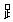\$BB-(B7d0.1
\$B5w(B7d5.8
\$BA)(B7d6.1    \$BD7(B7d6.3    \$BO)(B7d6.5    \$B@W(B7d6.7
\$BMY(B7d7.2
\$BF'(B7d8.3
\$BLv(B7d14.2
\$BFS(B
\$BG[(B7e3.2    \$B<`(B7e3.3
\$B?l(B7e4.3
\$B?](B7e5.3
\$B9Z(B7e6.1    \$B=7(B7e6.2    \$BMo(B7e6.4
\$B9s(B7e7.1    \$B;@(B7e7.2
\$B=9(B7e10.1
\$B>z(B7e13.1
\$B6b(B
\$B6b(B8a0.1
\$B?K(B8a2.3
\$BD`(B8a3.5
\$BF_(B8a4.2
\$BH-(B8a5.4    \$BE4(B8a5.6    \$BNk(B8a5.11   \$B1t(B8a5.14
\$B9[(B8a5.15
\$BA,(B8a6.1    \$B6d(B8a6.3    \$BLC(B8a6.4    \$BA-(B8a6.6
\$B=F(B8a6.9    \$BF<(B8a6.12
\$BCr(B8a7.2    \$B1T(B8a7.12
\$B?n(B8a8.2    \$BO#(B8a8.3    \$B:x(B8a8.10   \$B>{(B8a8.12
\$BO?(B8a8.16   \$B9](B8a8.20
\$BCC(B8a9.5
\$B:?(B8a10.2   \$BDC(B8a10.6
\$B6@(B8a11.6
\$B>b(B8a12.6
\$B4U(B8a15.2
\$B?)(B
\$B?)(B8b0.1
\$B52(B8b2.1
\$B0{(B8b4.1    \$BHS(B8b4.5
\$BK0(B8b5.1    \$B>~(B8b5.3    \$B;t(B8b5.4
\$B2n(B8b7.1
\$B4[(B8b8.3
\$Bp2(B
\$B@I(B8c2.1
\$BM:(B8c4.1    \$B=8(B8c4.2    \$B>G(B8c4.3
\$B2m(B8c5.1
\$B;s(B8c6.1    \$B;((B8c6.2    \$BC%(B8c6.4
\$BFq(B8c10.2   \$BN%(B8c10.3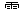\$B1+(B8d0.1
\$B@c(B8d3.2
\$B1@(B8d4.1    \$BJ7(B8d4.2
\$BMk(B8d5.1    \$BEE(B8d5.2    \$BNm(B8d5.4
\$B<{(B8d6.1
\$BNn(B8d7.2    \$B?L(B8d7.3
\$BAz(B8d9.2
\$BL8(B8d11.1
\$BO*(B8d13.1
\$BLg(B
\$BLg(B8e0.1
\$BLd(B8e3.1    \$BJD(B8e3.3
\$B4W(B8e4.2    \$B4V(B8e4.3    \$B3+(B8e4.6
\$BJ9(B8e6.1    \$BH6(B8e6.2    \$B3U(B8e6.3    \$B4X(B8e6.7
\$B1\(B8e7.2
\$BF.(B8e10.2
\$BJG(B
\$BD:(B9a2.1
\$B9`(B9a3.1    \$B=g(B9a3.2
\$BHR(B9a4.3    \$BMB(B9a4.5    \$B4h(B9a4.6
\$BNN(B9a5.2
\$BMj(B9a7.1    \$BF,(B9a7.6
\$BIQ(B9a8.2
\$BN`(B9a9.1    \$B4i(B9a9.3    \$B82(B9a9.5    \$B3[(B9a9.6
\$BBj(B9a9.7
\$B4j(B9a10.2
\$B8\(B9a12.2
\$BGO(B
\$BGO(B10a0.1
\$BBL(B10a4.1   \$B1X(B10a4.4   \$B6n(B10a4.5
\$BCs(B10a5.2
\$B53(B10a8.3   \$B83(B10a8.4   \$BA{(B10a8.5
\$B6C(B10a12.4
\$B5{(B
\$B5{(B11a0.1
\$BA/(B11a6.7
\$B7_(B11a8.9
\$BD;(B
\$BD;(B11b0.1
\$B7\(B11b8.4
```

Jim Kingdon, \$BF|K\8l(B, \$B3F.

I disclaim copyright interest in this page. You are encouraged but not required to preserve the web address http://www.panix.com/~kingdon/jp-ref.html when reproducing it. The bulk of this page was originally automatically produced from kanjidic by a script that I wrote. I doubt that it would be considered a derived work of kanjidic or of Spahn & Hadamitzky, but if the legal status is important better check on that issue.

Jim KINGDON: \$BF|K\8l(B English.